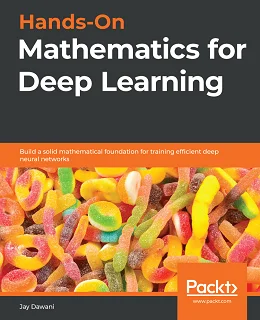# Hands-On Mathematics for Deep Learning### eBook Details:

• Paperback: 364 pages
• Publisher: WOW! eBook (June 12, 2020)
• Language: English
• ISBN-10: 1838647295
• ISBN-13: 978-1838647292

### eBook Description:

Hands-On Mathematics for Deep Learning: A comprehensive guide to getting well-versed with the mathematical techniques for building modern deep learning architectures

Most programmers and data scientists struggle with mathematics, having either overlooked or forgotten core mathematical concepts. This book uses Python libraries to help you understand the math required to build deep learning (DL) models.

You’ll begin by learning about core mathematical and modern computational techniques used to design and implement DL algorithms. This book will cover essential topics, such as linear algebra, eigenvalues and eigenvectors, the singular value decomposition concept, and gradient algorithms, to help you understand how to train deep neural networks. Later chapters focus on important neural networks, such as the linear neural network and multilayer perceptrons, with a primary focus on helping you learn how each model works. As you advance, you will delve into the math used for regularization, multi-layered DL, forward propagation, optimization, and backpropagation techniques to understand what it takes to build full-fledged DL models. Finally, you’ll explore CNN, recurrent neural network (RNN), and GAN models and their application.

• Understand the key mathematical concepts for building neural network models
• Discover core multivariable calculus concepts
• Improve the performance of deep learning models using optimization techniques